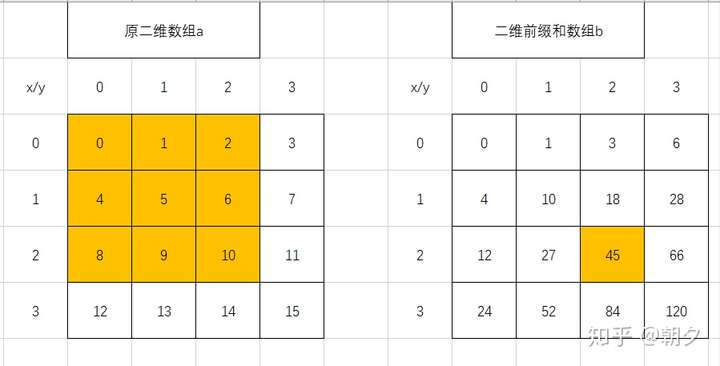# 算法基础-前缀和

## 一、什么是前缀和？## 二、如何得到前缀和？

for(int i=0;i<n;i++)
{
if(i==0) y[i]=x[i];
else y[i]=y[i-1]+x[i];
}for(int y=0;y<n;y++)//n行
for(int x=0;x<m;x++)//m列
{
if(x==0&&y==0) b[y][x]=a[y][x];//左上角的值
else if(x==0) b[y][x]=b[y-1][x]+a[y][x];//第一列
else if(y==0) b[y][x]=b[y][x-1]+a[y][x];//第一行
else b[y][x]=b[y-1][x]+b[y][x-1]-b[y-1][x-1]+a[y][x];
}



## 三、前缀和有什么用？

### 3.1 说明，其中是给定的区间。每次询问可直接输出答案，这样时间复杂度就降到了### 3.2.1 成绩百分比 改编自网易笔试题

(，成绩)。，其中表示分数，表示考到该分数的人数。

# 算法